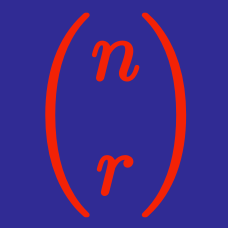Algebra

# Definition of Binomial Coefficient

Evaluate $\binom{8}{3}$.

Evaluate ${44 \choose 42}.$

What is the value of $n$ if ${n \choose 3}=35?$

What value of $n$ satisfies ${n \choose 2}=528?$

5 people became friends at a Summer Camp. On the last day, they shook each other's hands to say farewell. How many handshakes were there?

×